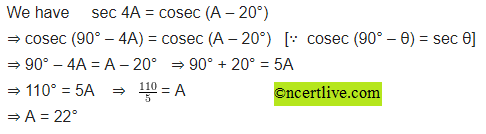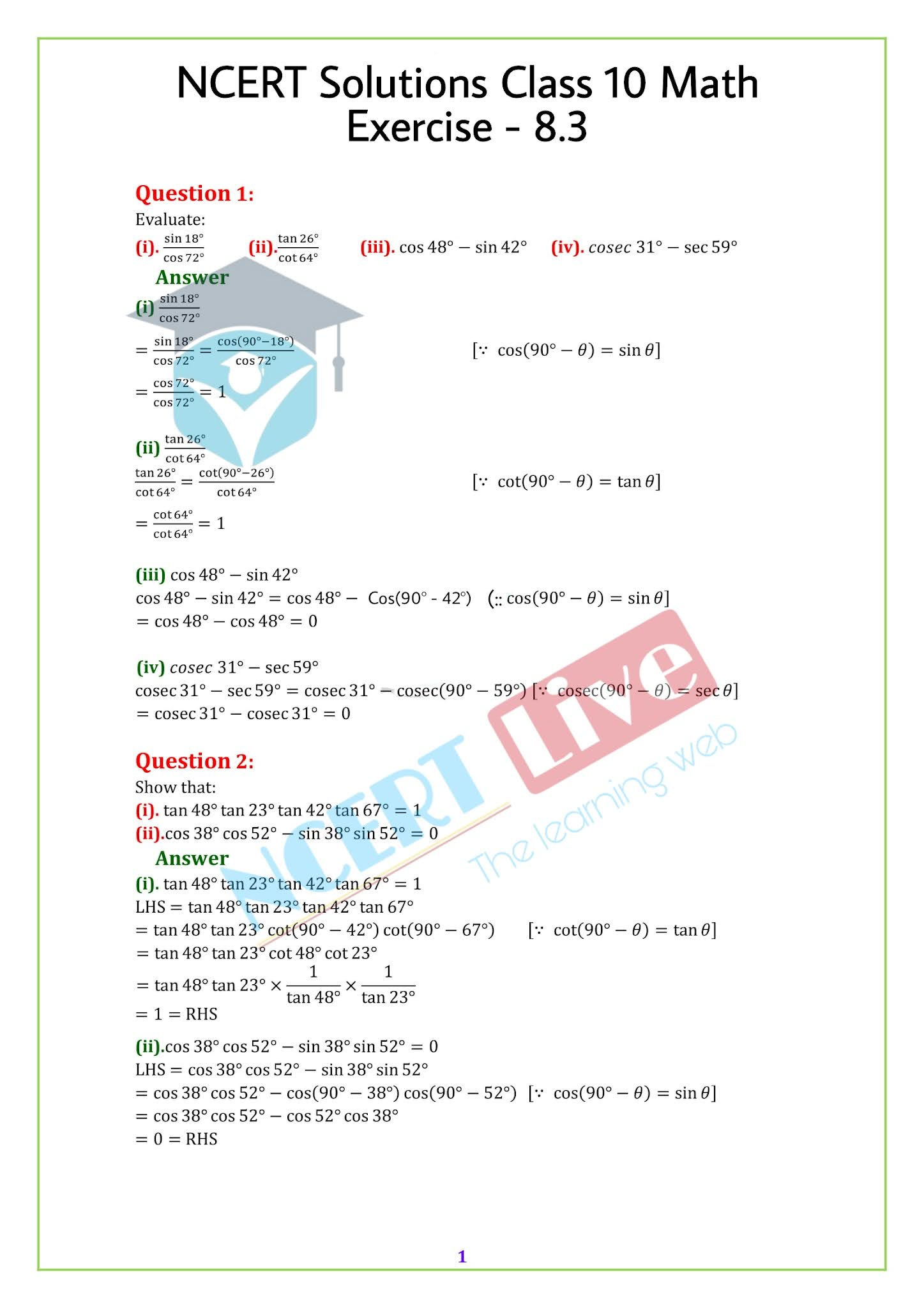NCERT solutions for Class 10 Maths Chapter 8, Introduction to Trigonometry, Exercise 8.3, is available here with all the solved problems from the NCERT textbook. The solutions are available in PDF format and it can be downloaded easily. The NCERT solutions are created by our Maths experts with proper geometric figures and explanations in a step by step procedure

 Exercise 8.1 Solutions Exercise 8.2 Solutions   English   or   Hindi Exercise 8.3 Solutions Exercise 8.4 Solutions   English   or   Hindi

NCERT Solution Exercise 8.3 Class 10

Exercise 8.3 Class 10  Question 1 Hindi
Q 1. निम्नलिखित का मान ज्ञात करो

Exercise 8.3 Class 10 Maths Question 2 Hindi
दर्शाइए की
(i) tan 48° tan 23° tan 42° tan 67° = 1
(ii) cos 38° cos 52° – sin 38° sin 52° = 0
Solution:Exercise 8.3 Class 10 Maths Question 3
Hindi
यदि tan 2A = cot (A – 18°), यह 2A एक न्यून कोण है ,तो A क्या होगा ?
Solution: Exercise 8.3 Class 10 Maths Question 4.
यदि tan A = cot B, सिद्ध करो की A + B = 90° है।
Solution:Exercise 8.3 Class 10 Maths Question 5
Hindi
यदि  4A = cosec (A – 20°), यह 4A एक न्यून कोण है ,तो A क्या होगा ?
Solutio
Hindi
यदि  A, B and C एक त्रिभुज ABC के आंतरिक कोण है तो दर्शाओ की sin(B+C/2) = cos A/2

A2
Solution:
Exercise 8.3 Class 10 Maths Question 7
Hindi
sin 61° + cos 75° को त्रिकोणमितीय अनुपात में दर्शाएं यदि कोण 0° and 45°के ,मध्य है।
Solution:Exercise 8.3 Class 10 Math PDF

Exercise 8.3 Class 10 Maths Question 1.
Evaluate:

Exercise 8.3 Class 10 Maths Question 2.
Show that:
(i) tan 48° tan 23° tan 42° tan 67° = 1
(ii) cos 38° cos 52° – sin 38° sin 52° = 0
Solution:Exercise 8.3 Class 10 Maths Question 3.
If tan 2A = cot (A – 18°), where 2A is an acute angle, find the value of A.
Solution: Exercise 8.3 Class 10 Maths Question 4.
If tan A = cot B, prove that A + B = 90°.
Solution:Exercise 8.3 Class 10 Maths Question 5.
If sec 4A = cosec (A – 20°), where 4A is an acute angle, find the value of A.
Solution:Exercise 8.3 Class 10 Maths Question 6.
If A, B and C are interior angles of a triangle ABC, then show that: sin(B+C/2) = cos A/2

A2
Solution:
Exercise 8.3 Class 10 Maths Question 7.
Express sin 61° + cos 75° in terms of trigonometric ratios of angles between 0° and 45°.
Solution:NCERT Solutions for Class 10 Maths

Chapter 1 Real Numbers
Chapter 2 Polynomials
Chapter 3 Pair of Linear Equations in Two Variables